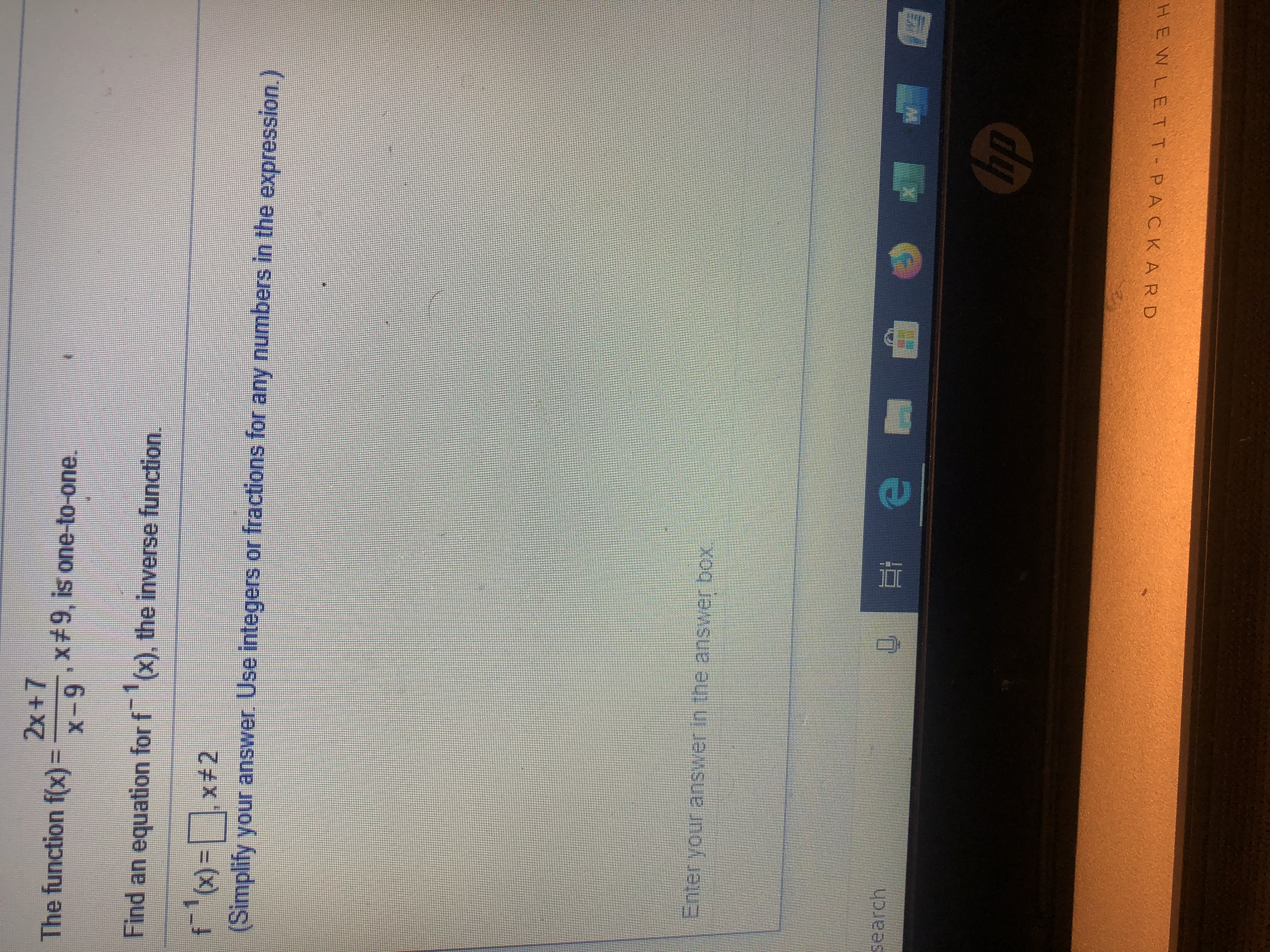# 2x+7The function x-9 X#9, is one-to-one.Find an equation for f (x), the inverse function.0)x2(Simplify your answer. Use integers or fractions for any numbers in the expression.)Enter your answer in the answer boxEeHearchhpHEWLETT PACK ARD

Question
15 viewshelp_outlineImage Transcriptionclose2x+7 The function x-9 X#9, is one-to-one. Find an equation for f (x), the inverse function. 0)x2 (Simplify your answer. Use integers or fractions for any numbers in the expression.) Enter your answer in the answer box Ee Hearch hp HEWLETT PACK ARD fullscreen
check_circle

Step 1

Consider the given one-to-one function:

Step 2

Now, to find the inverse, solve for x...

### Want to see the full answer?

See Solution

#### Want to see this answer and more?

Solutions are written by subject experts who are available 24/7. Questions are typically answered within 1 hour.*

See Solution
*Response times may vary by subject and question.
Tagged in
MathAlgebra

### Other